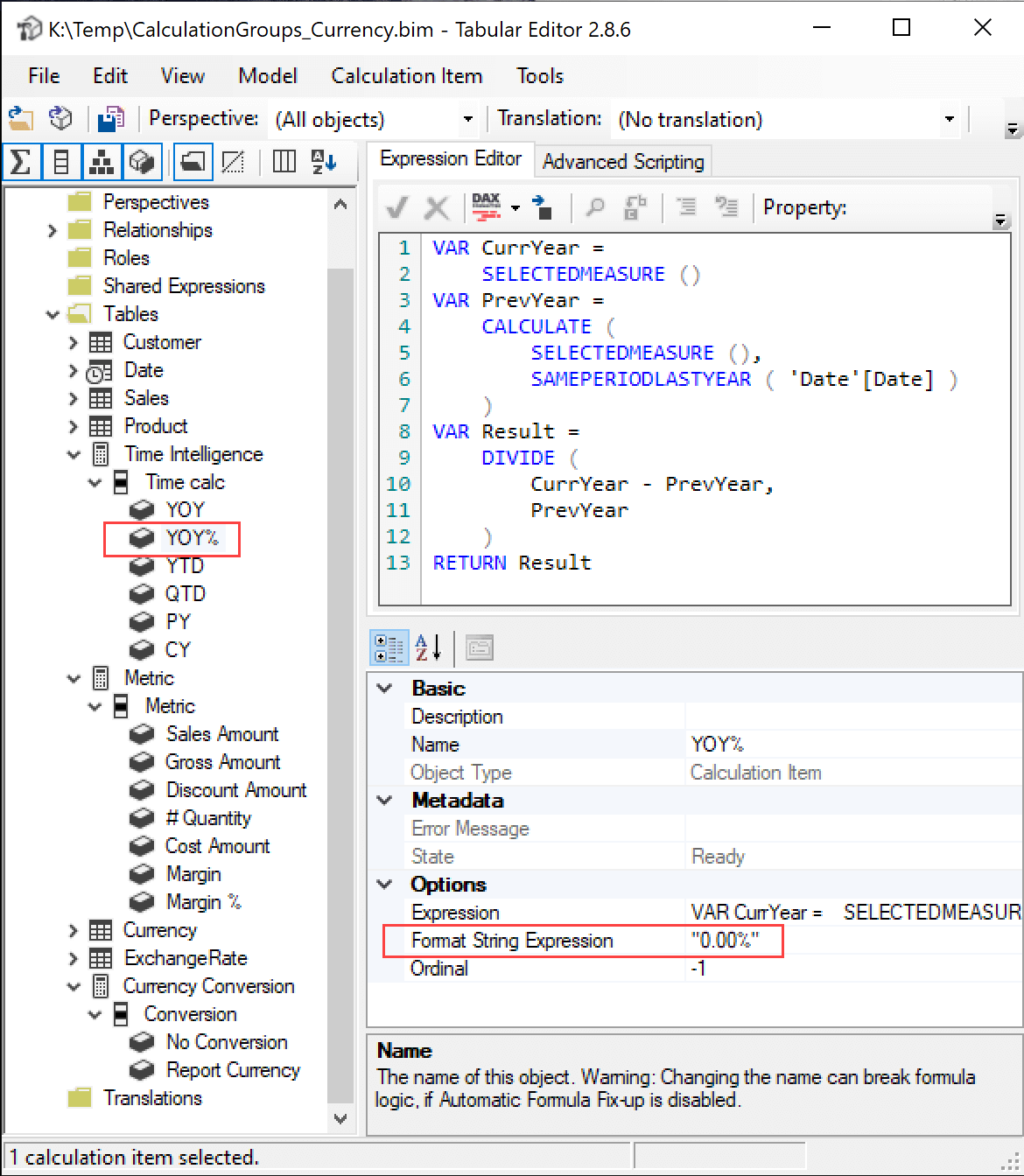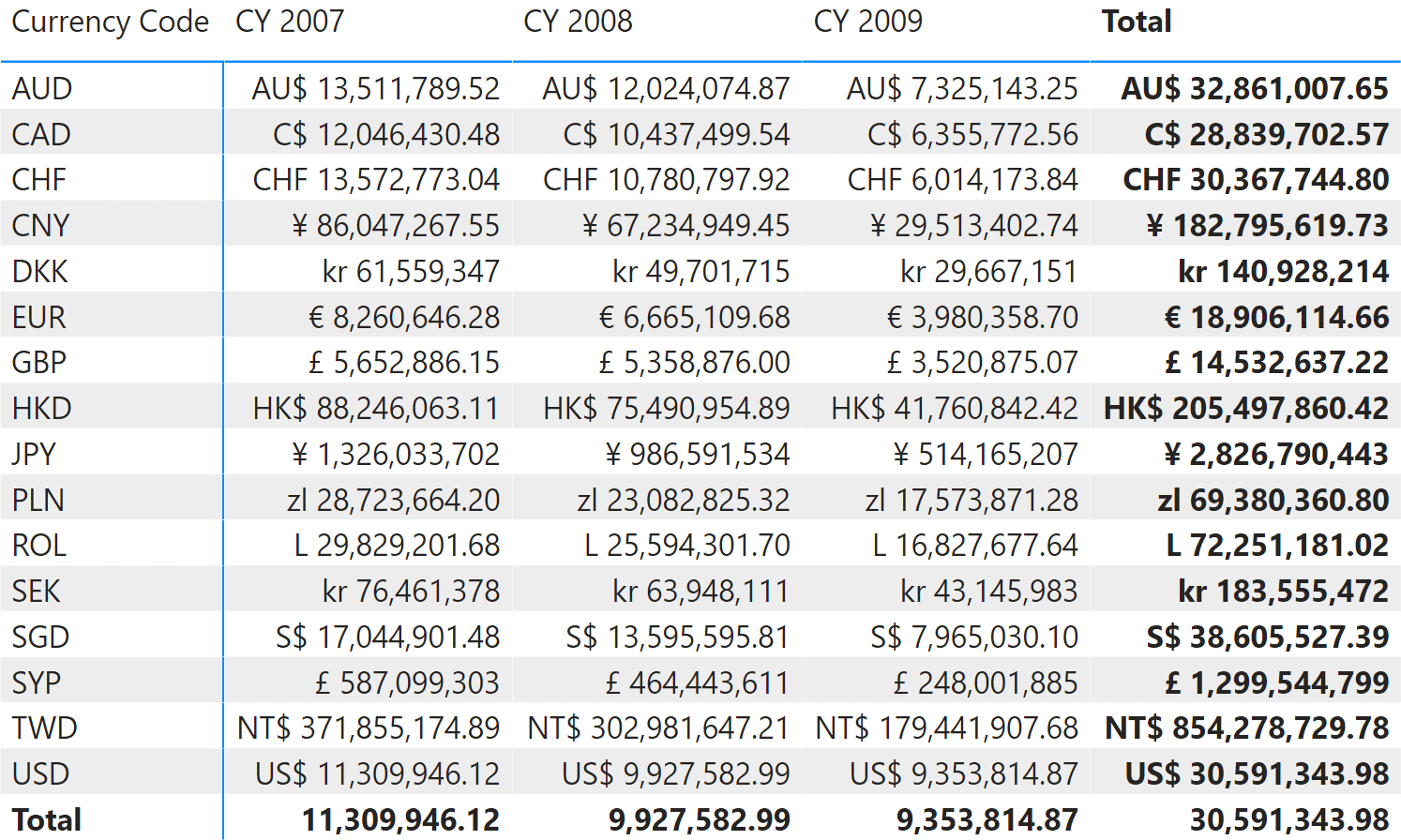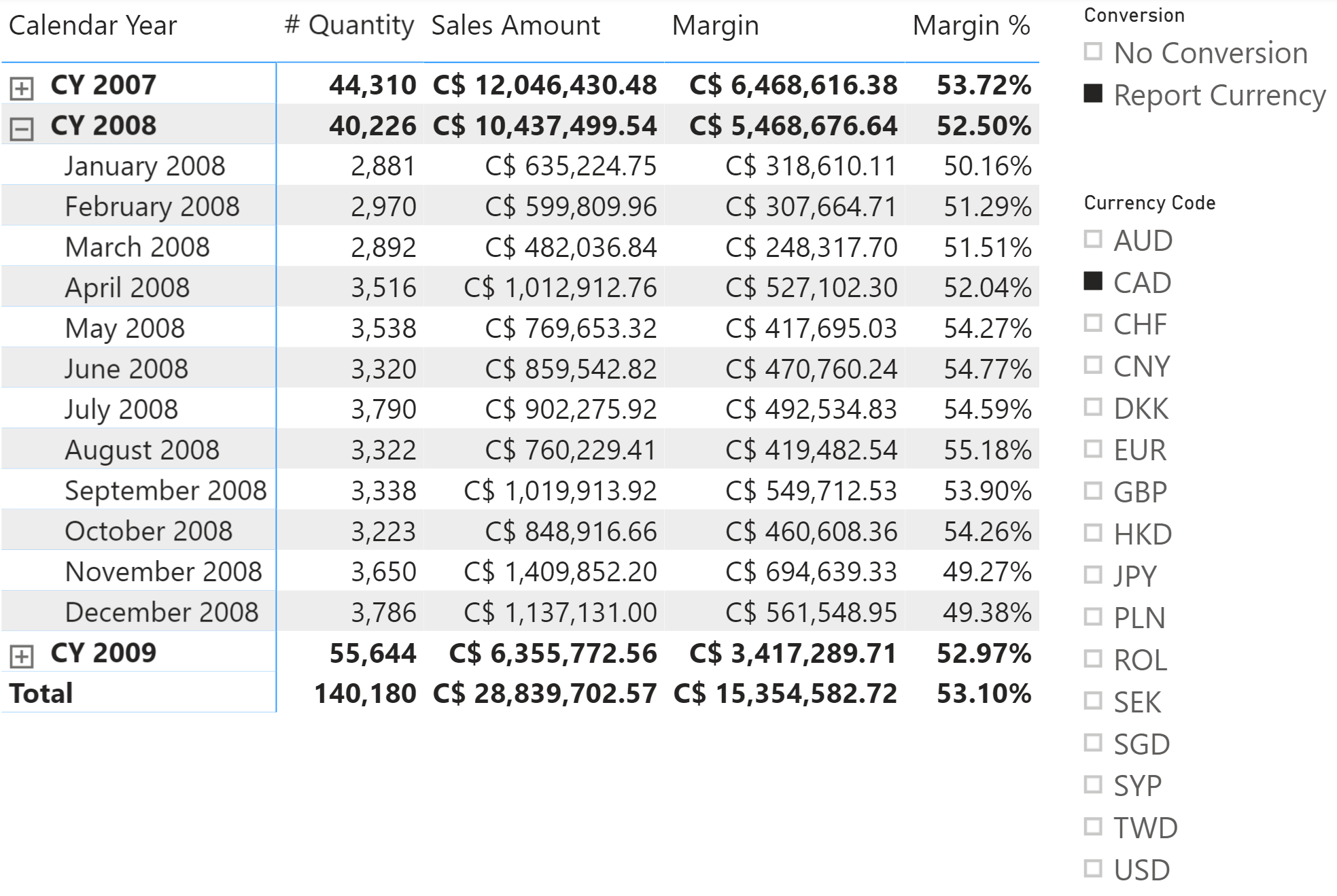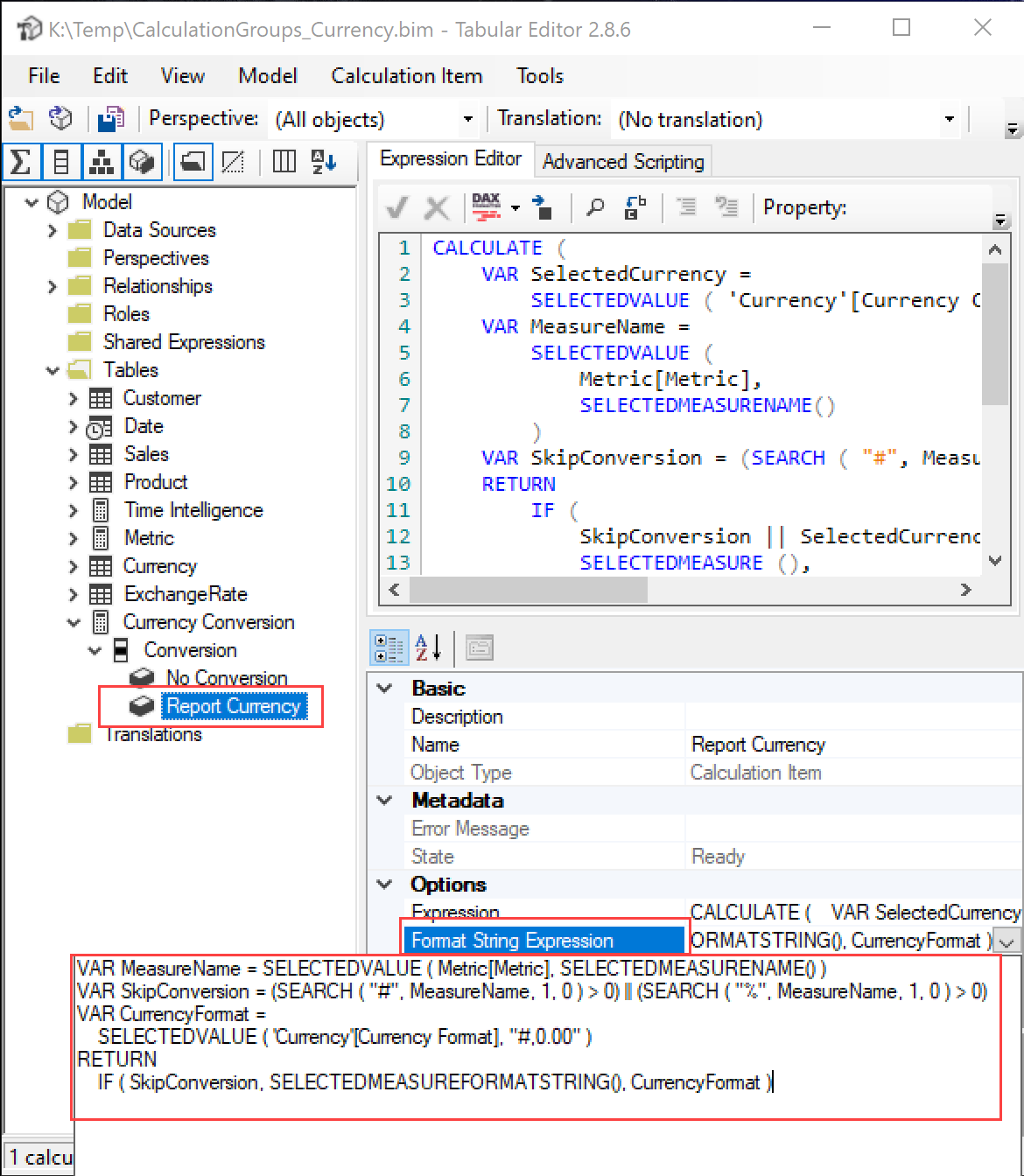UPDATE 2021-03-08: The original article had a mistake in precedence order of Time Intelligence and Currency Conversion calculation groups, that have been now inverted. The sample file has been updated as well.

Each calculation item can change the result of a measure through the DAX code in the Expression property. The author of a calculation item can also control the display format of the result, overwriting the standard behavior of the measure where the calculation item is applied. The Format String Expression property can contain a DAX expression that returns the format string to use after applying a calculation item to a measure reference.

For example, the dynamic format string can display the result of the YOY% calculation item as a percentage – that is the year-over-year as a percentage.In this specific example, we are looking at sales volumes. The Format String Expression property of the YOY% calculation item is always a percentage, regardless of the original format of the underlying measure.The model in this example has a Currency Conversion calculation group that applies a currency conversion exchange to all the measures representing currency amounts. The details of the calculation are not important for the purpose of this article. Instead, we focus on the goal of displaying the converted amount by using a different format for each currency available in the report.The conversion should not affect measures that are not displaying sales values. For example, in the following report only the Sales Amount and Margin measures get the currency conversion and the corresponding format, whereas # Quantity and Margin % are not affected by the Currency Conversion calculation group.The Report Currency calculation item applies a custom format string depending on the selected currency, checking whether the measure selected should be converted or not. This is done by the DAX expression included in the Format String Expression property.The complete code of the Format String Expression property is the following:

```VAR MeasureName =
SELECTEDVALUE ( Metric[Metric], SELECTEDMEASURENAME () )
VAR SkipConversion =
( SEARCH ( "#", MeasureName, 1, 0 ) > 0 )
|| ( SEARCH ( "%", MeasureName, 1, 0 ) > 0 )
VAR CurrencyFormat =
SELECTEDVALUE ( 'Currency'[Currency Format], "#,0.00" )
RETURN
IF (
SkipConversion,
SELECTEDMEASUREFORMATSTRING (),
CurrencyFormat
)
```

The MeasureName variable retrieves the measure selected by looking at the Metric[Metric] selection, because the Metric selection can override the measure selected in the report. If the Metric calculation group is not used or does not have a valid selection, then the SELECTEDMEASURENAME function returns the name of the active measure.

The SkipConversion variable controls whether the measure should be converted based on the measure name.

The CurrencyFormat variable retrieves the format string from the corresponding column in the Currency table, where only one value should be selected to ensure the currency conversion can take place. In case the currency conversion is not needed, the SELECTEDMEASUREFORMATSTRING function returns the format string of the current measure. This format string may already have been defined by another calculation item with higher precedence.

## The importance of the precedence order

There are three calculation groups in the model used in this example, with the following precedence order:

• Metric: 10
• Currency Conversion: 20
• Time Intelligence: 30

The calculation group with the highest precedence order is applied first. This is valid for both the Expression and the Format String Expression properties of calculation items.

This is important considering how the MeasureName variable is evaluated in the Report Currency calculation item. Because Report Currency has a higher precedence, it is applied to the measure reference when the Metric calculation group is still in the filter context and has not yet been applied. For this reason it is possible to retrieve the current selection of Metric[Metric] from the filter context. In this case this technique is required to make sure that the behavior of the Report Currency calculation item is consistent regardless of the technique used to choose the measure to display in a report: both direct measure selection or Metric calculation group selection should work seamlessly.

## Conclusions

The Format String Expression property enables the control of the format string of any measure in a dynamic way. The SELECTEDMEASUREFORMATSTRING function allows access to the existing format string – that could be the one originally defined by the measure or the one already modified by another calculation item. Controlling the format string is vital to provide a good user experience when using calculation items that can change the numeric representation of the result of a measure.

SELECTEDMEASURENAMEReturns name of the measure that is currently being evaluated.

`SELECTEDMEASURENAME ( )`

SELECTEDMEASUREFORMATSTRINGReturns format string for the measure that is currently being evaluated.

`SELECTEDMEASUREFORMATSTRING ( )`